Search by Topic

Resources tagged with Visualising similar to Decimal Time:

Filter by: Content type:
Age range:
Challenge level:

There are 176 results

Broad Topics > Using, Applying and Reasoning about Mathematics > Visualising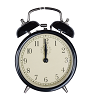On Time

Age 11 to 14 Challenge Level:

On a clock the three hands - the second, minute and hour hands - are on the same axis. How often in a 24 hour day will the second hand be parallel to either of the two other hands?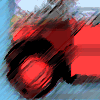Buses

Age 11 to 14 Challenge Level:

A bus route has a total duration of 40 minutes. Every 10 minutes, two buses set out, one from each end. How many buses will one bus meet on its way from one end to the other end?Circuit Training

Age 14 to 16 Challenge Level:

Mike and Monisha meet at the race track, which is 400m round. Just to make a point, Mike runs anticlockwise whilst Monisha runs clockwise. Where will they meet on their way around and will they ever. . . .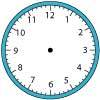Right Time

Age 11 to 14 Challenge Level:

At the time of writing the hour and minute hands of my clock are at right angles. How long will it be before they are at right angles again?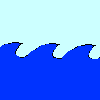Crossing the Atlantic

Age 11 to 14 Challenge Level:

Every day at noon a boat leaves Le Havre for New York while another boat leaves New York for Le Havre. The ocean crossing takes seven days. How many boats will each boat cross during their journey?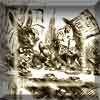A Problem of Time

Age 14 to 16 Challenge Level:

Consider a watch face which has identical hands and identical marks for the hours. It is opposite to a mirror. When is the time as read direct and in the mirror exactly the same between 6 and 7?Konigsberg Plus

Age 11 to 14 Challenge Level:

Euler discussed whether or not it was possible to stroll around Koenigsberg crossing each of its seven bridges exactly once. Experiment with different numbers of islands and bridges.One and Three

Age 14 to 16 Challenge Level:

Two motorboats travelling up and down a lake at constant speeds leave opposite ends A and B at the same instant, passing each other, for the first time 600 metres from A, and on their return, 400. . . .Tessellating Hexagons

Age 11 to 14 Challenge Level:

Which hexagons tessellate?Around and Back

Age 14 to 16 Challenge Level:

A cyclist and a runner start off simultaneously around a race track each going at a constant speed. The cyclist goes all the way around and then catches up with the runner. He then instantly turns. . . .Clocked

Age 11 to 14 Challenge Level:

Is it possible to rearrange the numbers 1,2......12 around a clock face in such a way that every two numbers in adjacent positions differ by any of 3, 4 or 5 hours?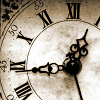John's Train Is on Time

Age 11 to 14 Challenge Level:

A train leaves on time. After it has gone 8 miles (at 33mph) the driver looks at his watch and sees that the hour hand is exactly over the minute hand. When did the train leave the station?Königsberg

Age 11 to 14 Challenge Level:

Can you cross each of the seven bridges that join the north and south of the river to the two islands, once and once only, without retracing your steps?Flight of the Flibbins

Age 11 to 14 Challenge Level:

Blue Flibbins are so jealous of their red partners that they will not leave them on their own with any other bue Flibbin. What is the quickest way of getting the five pairs of Flibbins safely to. . . .Problem Solving, Using and Applying and Functional Mathematics

Age 5 to 18 Challenge Level:

Problem solving is at the heart of the NRICH site. All the problems give learners opportunities to learn, develop or use mathematical concepts and skills. Read here for more information.Hidden Rectangles

Age 11 to 14 Challenge Level:

Rectangles are considered different if they vary in size or have different locations. How many different rectangles can be drawn on a chessboard?Rolling Around

Age 11 to 14 Challenge Level:

A circle rolls around the outside edge of a square so that its circumference always touches the edge of the square. Can you describe the locus of the centre of the circle?Tourism

Age 11 to 14 Challenge Level:

If you can copy a network without lifting your pen off the paper and without drawing any line twice, then it is traversable. Decide which of these diagrams are traversable.Frogs

Age 11 to 14 Challenge Level:

How many moves does it take to swap over some red and blue frogs? Do you have a method?Coloured Edges

Age 11 to 14 Challenge Level:

The whole set of tiles is used to make a square. This has a green and blue border. There are no green or blue tiles anywhere in the square except on this border. How many tiles are there in the set?Painted Cube

Age 14 to 16 Challenge Level:

Imagine a large cube made from small red cubes being dropped into a pot of yellow paint. How many of the small cubes will have yellow paint on their faces?Picturing Square Numbers

Age 11 to 14 Challenge Level:

Square numbers can be represented as the sum of consecutive odd numbers. What is the sum of 1 + 3 + ..... + 149 + 151 + 153?Picturing Triangular Numbers

Age 11 to 14 Challenge Level:

Triangular numbers can be represented by a triangular array of squares. What do you notice about the sum of identical triangle numbers?An Unusual Shape

Age 11 to 14 Challenge Level:

Can you maximise the area available to a grazing goat?Age 11 to 14 Challenge Level:

Four rods, two of length a and two of length b, are linked to form a kite. The linkage is moveable so that the angles change. What is the maximum area of the kite?Convex Polygons

Age 11 to 14 Challenge Level:

Show that among the interior angles of a convex polygon there cannot be more than three acute angles.Dotty Triangles

Age 11 to 14 Challenge Level:

Imagine an infinitely large sheet of square dotty paper on which you can draw triangles of any size you wish (providing each vertex is on a dot). What areas is it/is it not possible to draw?Pattern Power

Age 5 to 14

Mathematics is the study of patterns. Studying pattern is an opportunity to observe, hypothesise, experiment, discover and create.Framed

Age 11 to 14 Challenge Level:

Seven small rectangular pictures have one inch wide frames. The frames are removed and the pictures are fitted together like a jigsaw to make a rectangle of length 12 inches. Find the dimensions of. . . .Tetra Square

Age 11 to 14 Challenge Level:

ABCD is a regular tetrahedron and the points P, Q, R and S are the midpoints of the edges AB, BD, CD and CA. Prove that PQRS is a square.Take Ten

Age 11 to 14 Challenge Level:

Is it possible to remove ten unit cubes from a 3 by 3 by 3 cube so that the surface area of the remaining solid is the same as the surface area of the original?AMGM

Age 14 to 16 Challenge Level:

Can you use the diagram to prove the AM-GM inequality?Hypotenuse Lattice Points

Age 14 to 16 Challenge Level:

The triangle OMN has vertices on the axes with whole number co-ordinates. How many points with whole number coordinates are there on the hypotenuse MN?Squares, Squares and More Squares

Age 11 to 14 Challenge Level:

Can you dissect a square into: 4, 7, 10, 13... other squares? 6, 9, 12, 15... other squares? 8, 11, 14... other squares?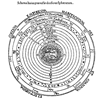Shaping the Universe II - the Solar System

Age 11 to 16

The second in a series of articles on visualising and modelling shapes in the history of astronomy.Jam

Age 14 to 16 Challenge Level:

A game for 2 playersCoordinate Patterns

Age 11 to 14 Challenge Level:

Charlie and Alison have been drawing patterns on coordinate grids. Can you picture where the patterns lead?Sliding Puzzle

Age 11 to 16 Challenge Level:

The aim of the game is to slide the green square from the top right hand corner to the bottom left hand corner in the least number of moves.Air Nets

Age 7 to 18 Challenge Level:

Can you visualise whether these nets fold up into 3D shapes? Watch the videos each time to see if you were correct.Seven Squares

Age 11 to 14 Challenge Level:

Watch these videos to see how Phoebe, Alice and Luke chose to draw 7 squares. How would they draw 100?Route to Infinity

Age 11 to 14 Challenge Level:

Can you describe this route to infinity? Where will the arrows take you next?Steel Cables

Age 14 to 16 Challenge Level:

Some students have been working out the number of strands needed for different sizes of cable. Can you make sense of their solutions?3D Stacks

Age 7 to 14 Challenge Level:

Can you find a way of representing these arrangements of balls?Auditorium Steps

Age 7 to 14 Challenge Level:

What is the shape of wrapping paper that you would need to completely wrap this model?Polygon Rings

Age 11 to 14 Challenge Level:

Join pentagons together edge to edge. Will they form a ring?Troublesome Dice

Age 11 to 14 Challenge Level:

When dice land edge-up, we usually roll again. But what if we didn't...?Partly Painted Cube

Age 14 to 16 Challenge Level:

Jo made a cube from some smaller cubes, painted some of the faces of the large cube, and then took it apart again. 45 small cubes had no paint on them at all. How many small cubes did Jo use?Christmas Chocolates

Age 11 to 14 Challenge Level:

How could Penny, Tom and Matthew work out how many chocolates there are in different sized boxes?Mystic Rose

Age 14 to 16 Challenge Level:

Use the animation to help you work out how many lines are needed to draw mystic roses of different sizes.Speeding Boats

Age 14 to 16 Challenge Level:

Two boats travel up and down a lake. Can you picture where they will cross if you know how fast each boat is travelling?